# Glasses

Imagine a set of students in your class (number of students: 19), who wears glasses. How much minimum and maximum element may contain this set.

min =  0
max =  19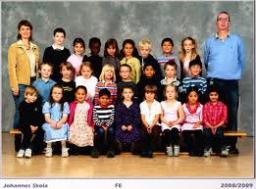We will be pleased if You send us any improvements to this math problem. Thank you!## Related math problems and questions:

• Trousers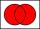In the class was 12 students. Nine students wearing trousers and turtleneck eight. How many students worn trousers with a turtleneck?
• Camp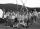In a class are 26 children. During the holidays 16 children were in the camps and 14 children on holiday with their parents. Determine the minimum and maximum number of children that may have been in the camp and on holiday with their parents at the same
• Subsets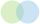How many 19 element's subsets can be made from the 26 element set?
• Glasses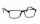There are 36 pupils in the class. Nine girls wear glasses. Boys with glasses are five less than girls without glasses. Boys without glasses are two times more than girls without glasses. How many boys and how many girls?
• News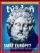From 28 students buy magazine 10 The economist students, 10 Pravda students and 18 students don't buy any of these magazines. How many students buy both magazines?
• Class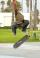The class has 18 students. Everyone knows inline skating or skateboarding. Inline skating can ride 11 students on a skateboard 10. How many ride on inline skates and on skateboard?
• Ten boys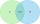Ten boys chose to go to the supermarket. Six boys bought gum and nine boys bought a lollipop. How many boys bought gum and a lollipop?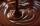In the box were a total of 200 cookies. These products have sugar and chocolate topping. The chocolate topping is used on 157 cookies. Sugar topping is used on 100 cakes. How many of these cookies have two frostings?
• Two sets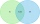Suppose Set B contains 69 elements and the total number elements in either Set A or Set B is 124. If the Sets A and B have 29 elements in common, how many elements are contained in set A?
• Skoda carsThere were 16 passenger cars in the parking. It was the 10 blue and 10 Skoda cars. How many are blue Skoda cars in the parking?
• The average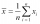The average of one set of 4 numbers is 35. The average of another set of number is 20. The average of the numbers in the two sets is 30. How many numbers are there in the other set?
• 5-number summaryGiven the following 5-number summary: 11, 19, 24, 30, 48 which of the statistics cannot be determined?
• Jane classWhen asked how many students are in class, Jane said, if we increase the number of students in our class by hundred % and then add half the number of students, we get 100. How many students are in Jane's class?
• Seating rules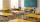In a class are 24 seats but in 7.B class are only 18 students. How many ways can students sit? (The class has 12 benches. A bench is for a pair of students.) Result (large number) logarithm and thus write down as powers of 10.
• Meanif the mean of the set of data 5, 17, 19, 14, 15, 17, 7, 11, 16, 19, 5, 5, 10, 8, 13, 14, 4, 2, 17, 11, x is -91.74, what is the value of x?
• Operations with sets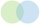The set B - A has twice as fewer elements than the set A - B and four times fewer elements than the set A ∩ B. How many times more elements does the set A have than the set B?
• School tripThe class has 19 students. What different ways can students be accommodated in the hostel, where available 3× 2-bed, 3× 3-bed and 1× 4-bed rooms? (Each room has its unique number)